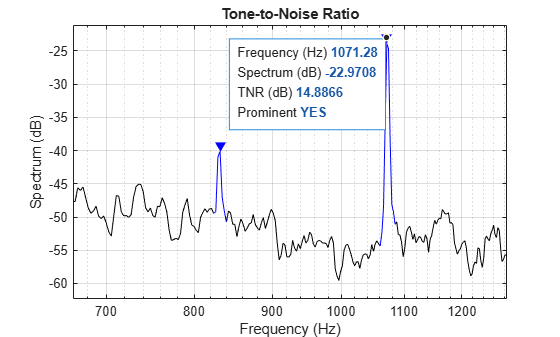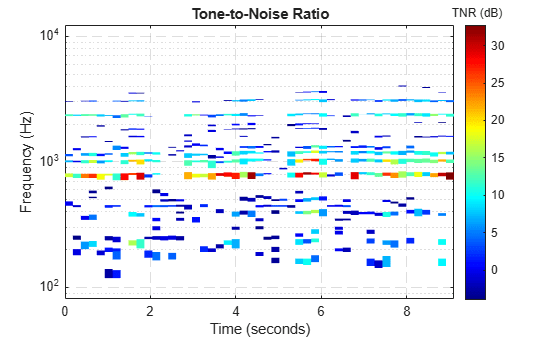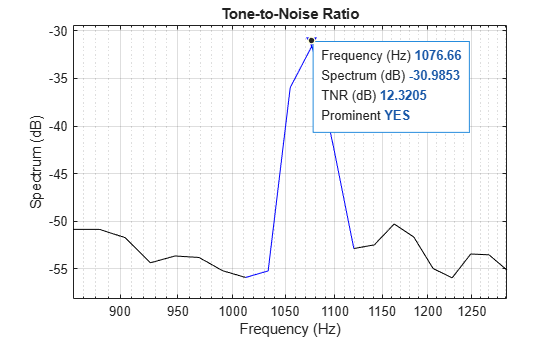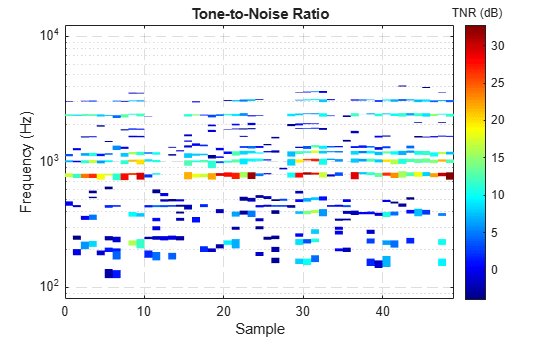# acousticToneToNoiseRatio

Identify and quantify tones in acoustic signals

Since R2023b

## Syntax

``[tnr,tnrFreq] = acousticToneToNoiseRatio(audioIn,fs)``
``[tnr,tnrFreq,isProminent] = acousticToneToNoiseRatio(audioIn,fs)``
``[tnr,tnrFreq,isProminent,timestamps] = acousticToneToNoiseRatio(audioIn,fs,TimeVarying=true)``
``[___] = acousticToneToNoiseRatio(___,Name=Value)``
``acousticToneToNoiseRatio(___)``

## Description

example

````[tnr,tnrFreq] = acousticToneToNoiseRatio(audioIn,fs)` detects tones in the audio and returns the corresponding tone-to-noise ratios (TNR) according to ECMA-418-1 .```

example

````[tnr,tnrFreq,isProminent] = acousticToneToNoiseRatio(audioIn,fs)` also returns a logical vector specifying which tones are prominent according to ECMA-418-1.```

example

````[tnr,tnrFreq,isProminent,timestamps] = acousticToneToNoiseRatio(audioIn,fs,TimeVarying=true)` detects tones and measures TNR in a time-varying signal. This syntax also returns a vector of timestamps for each time segment in the input signal.```

example

````[___] = acousticToneToNoiseRatio(___,Name=Value)` specifies options using one or more name-value arguments. For example, `acousticToneToNoiseRatio(x,fs,ReturnProminentOnly=true)` returns only the prominent tones detected in the input signal.```

example

````acousticToneToNoiseRatio(___)` with no output arguments plots the detected tones and their TNRs.```

## Examples

collapse all

Read in an audio signal containing the sound from a turbine.

`[x,fs] = audioread("Turbine-16-44p1-mono-22secs.wav");`

Listen to the first three seconds of the audio signal.

`sound(x(1:fs*3),fs)`

Use `acousticToneToNoiseRatio` to detect and measure tones in the stationary signal.

• `tnrFreq` contains the frequencies of the detected tones in Hz.

• `tnr` contains the tone-to-noise ratios of the detected tones.

`[tnr,tnrFreq] = acousticToneToNoiseRatio(x,fs);`

Call `acousticToneToNoiseRatio` with no output arguments to plot the tones. The plot contains data tips with information about the detected tones.

`acousticToneToNoiseRatio(x,fs)`Read in an audio signal containing a train whistle and listen to it.

```[x,fs] = audioread("TrainWhistle-16-44p1-mono-9secs.wav"); sound(x,fs)```

Call `acousticToneToNoiseRatio` with `TimeVarying` set to `true` to detect the tones in the time-varying signal.

`[tnr,tnrFreq,isProminent,timestamps] = acousticToneToNoiseRatio(x,fs,TimeVarying=true);`

Call `acousticToneToNoiseRatio` with no output arguments to plot the time-varying tones and their tone-to-noise ratios. The plot contains data tips with information about the detected tones.

`acousticToneToNoiseRatio(x,fs,TimeVarying=true)`Read in an audio signal containing the sound from a turbine.

`[x,fs] = audioread("Turbine-16-44p1-mono-22secs.wav");`

Use `acousticToneToNoiseRatio` to detect the tones in the signal. Specify the third output argument to get a logical vector indicating which tones are prominent.

`[tnr,tnrFreq,isProminent] = acousticToneToNoiseRatio(x,fs);`

Use `isProminent` to get the frequencies of the prominent tones. This signal has one prominent tone.

`tnrFreq(isProminent)`
```ans = 1.0713e+03 ```

Read in an audio signal containing the sound from a turbine.

`[x,fs] = audioread("Turbine-16-44p1-mono-22secs.wav");`

Specify custom parameters for the spectral analysis performed by `acousticToneToNoiseRatio`.

```nfft = 2048; window = hamming(nfft,"periodic"); overlap = round(0.75*numel(window)); freqRange = [100 16000];```

Call `acousticToneToNoiseRatio` with the custom parameters and see the resulting plot.

```acousticToneToNoiseRatio(x,fs,FFTLength=nfft,Window=window, ... OverlapLength=overlap,FrequencyRange=freqRange)```Read in an audio signal containing the sound from a turbine.

`[x,fs] = audioread("Turbine-16-44p1-mono-22secs.wav");`

Compute the magnitude spectrum of the stationary signal and convert it to dB.

```nfft = 16384; [pxx,f] = pwelch(x,hann(nfft,"periodic"),nfft/2,nfft,fs); pxxDB = 10*log10(pxx);```

Use `acousticToneToNoiseRatio` to detect and measure tones in the spectrum.

`acousticToneToNoiseRatio(pxxDB,f);`Read in an audio signal containing a train whistle.

`[x,fs] = audioread("TrainWhistle-16-44p1-mono-9secs.wav");`

Compute the spectrogram of the time-varying signal and convert it to dB.

```nfft = 16384; h = hann(nfft,"periodic"); [~,fs,~,psd] = spectrogram(x,h,nfft/2,nfft,fs); psdDB = 10*log10(psd);```

Use `acousticToneToNoiseRatio` to detect and measure tones in the spectrogram.

`acousticToneToNoiseRatio(psdDB,fs,TimeVarying=true)`## Input Arguments

collapse all

Audio input, specified as a column vector or matrix. The audio input can be a time-domain or frequency-domain signal.

• If `fs` is a scalar, `audioIn` must be a column vector, which `acousticToneToNoiseRatio` interprets as a single-channel time-domain signal.

• If `fs` is a vector and `TimeVarying` is `false` or unspecified, `audioIn` must be a column vector, which `acousticToneToNoiseRatio` interprets as a magnitude spectrum in dB.

• If `fs` is a vector and `TimeVarying` is `true`, `audioIn` must be a matrix, which `acousticToneToNoiseRatio` interprets as a magnitude spectrogram in dB. Each column in the spectrogram is a spectrum corresponding to a time segment.

Data Types: `single` | `double`

Sample rate or frequency vector in Hz, specified as a positive scalar or vector.

• If `fs` is a scalar, it is the sample rate of the time-domain input signal `audioIn`. To cover the frequency range specified in the ECMA-418-1  standard, the sample rate should be at least 32 kHz.

• If `fs` is a vector, it contains the frequencies corresponding to each row in the frequency-domain input signal `audioIn`.

Data Types: `single` | `double`

### Name-Value Arguments

Specify optional pairs of arguments as `Name1=Value1,...,NameN=ValueN`, where `Name` is the argument name and `Value` is the corresponding value. Name-value arguments must appear after other arguments, but the order of the pairs does not matter.

Before R2021a, use commas to separate each name and value, and enclose `Name` in quotes.

Example: ```acousticToneToNoiseRatio(x,fs,FrequencyRange=[500 2000])```

Time-varying input signal, specified as `true` or `false`. If `TimeVarying` is `true`, `acousticToneToNoiseRatio` detects and measures tones in each time segment of the time-varying signal.

• If the input is a time-domain signal, the `Window` and `OverlapLength` determine the time segments of the time-varying signal.

• If the input is a frequency-domain signal, each column in the magnitude spectrogram corresponds to a time segment.

Data Types: `logical`

FFT length, specified as a positive integer greater than or equal to the length of `Window`.

This argument does not apply if the input is a frequency-domain signal.

Data Types: `single` | `double`

Analysis window, specified as a vector.

This argument does not apply if the input is a frequency-domain signal.

Data Types: `single` | `double`

Overlap length, specified as a positive integer less than the length of `Window`. This argument specifies the number of samples overlap between adjacent windows.

This argument does not apply if the input is a frequency-domain signal.

Data Types: `single` | `double`

Frequency range in Hz over which to analyze tones, specified as a two-element row vector.

Data Types: `single` | `double`

Flag to only return prominent tones, specified as `true` or `false`. If you specify this argument as `true`, `acousticToneToNoiseRatio` returns only tones that are prominent according to the ECMA-418-1 standard .

Data Types: `logical`

Flag to combine proximal tones, specified as `true` or `false`. If this argument is unspecified or `true`, `acousticToneToNoiseRatio` combines tones that are close enough to be perceived as a single tone according to the proximity criteria of the ECMA-418-1 standard.

Data Types: `single` | `double`

## Output Arguments

collapse all

Tone-to-noise ratios (TNR) of detected tones, returned as a vector in decreasing order. The TNR is a metric that measures perceived tonality, and it is defined in the ECMA-418-1 standard . Each value in the vector is the TNR of the corresponding frequency in `tnrFreq`.

If `TimeVarying` is `true`, `acousticToneToNoiseRatio` returns `tnr` as a cell array of vectors where each cell corresponds to a time segment.

Frequencies of the detected tones in Hz, returned as a vector where each element corresponds to a TNR value in `tnr`.

If `TimeVarying` is `true`, `acousticToneToNoiseRatio` returns `tnrFreq` as a cell array of vectors where each cell corresponds to a time segment.

Prominent tones according to the ECMA-418-1 standard, returned as a logical vector with a value corresponding to each tone in `tnrFreq` indicating whether it is prominent.

If `TimeVarying` is `true`, `acousticToneToNoiseRatio` returns `isProminent` as a cell array of vectors where each cell corresponds to a time segment.

Timestamps of each time segment in the input signal in seconds, returned as a vector.

This output argument applies only if you set `TimeVarying` to `true`.

## Algorithms

The `acousticToneToNoiseRatio` function detects the tones in the input signal using the methods proposed in .

 Bray, Wade R. “Methods for Automating Prominent Tone Evaluation and for Considering Variations with Time or Other Reference Quantities.” The Journal of the Acoustical Society of America, vol. 123, no. 5, May 2008, pp. 3685–3685. DOI.org (Crossref), https://doi.org/10.1121/1.2935056.

 ECMA-418-1. "Psychoacoustic metrics for ITT equipment — Part 1 (prominent discrete tones)." Ecma International. https://www.ecma-international.org/publications-and-standards/standards/ecma-418/.